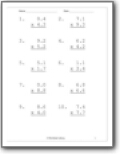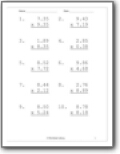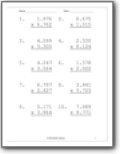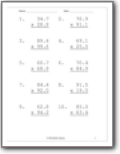# Decimal Multiplication Worksheets

#### How to Multiply Decimals

Decimals itself are complex but performing operations on them is a far difficult task. In some calculations, the problems become harder, if the basics aren't clear. So, there are a few tips which you must remember when you're multiplying decimals. First, you must keep in mind that multiplying decimals is the same as multiplying whole numbers. Always remember this concept. Place the decimal point in the result before taking the numbers' total sum after the decimal point. Multiply decimal numbers similar as you multiply whole numbers. Place the decimal point in the result before the total sum of decimal places.###### Tenths

Students will multiply two values that together that have a ones and tenths value.###### Hundredths Place

You will multiply and find the product of two values that have a ones, tenths, and hundredths value.###### Thousandths

Following this pattern, we looks determine the final product of values that include a thousandths place.###### Multiply Tens, Ones, and Tenths

You will multiply two values that have all these place values.